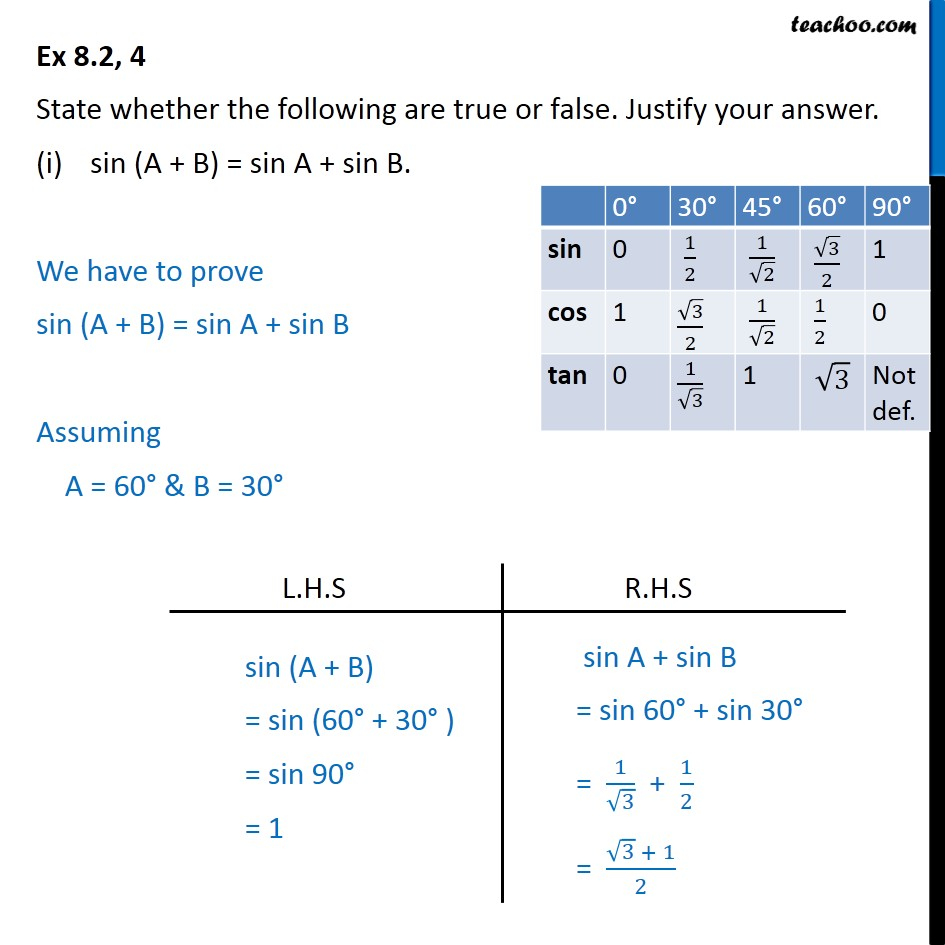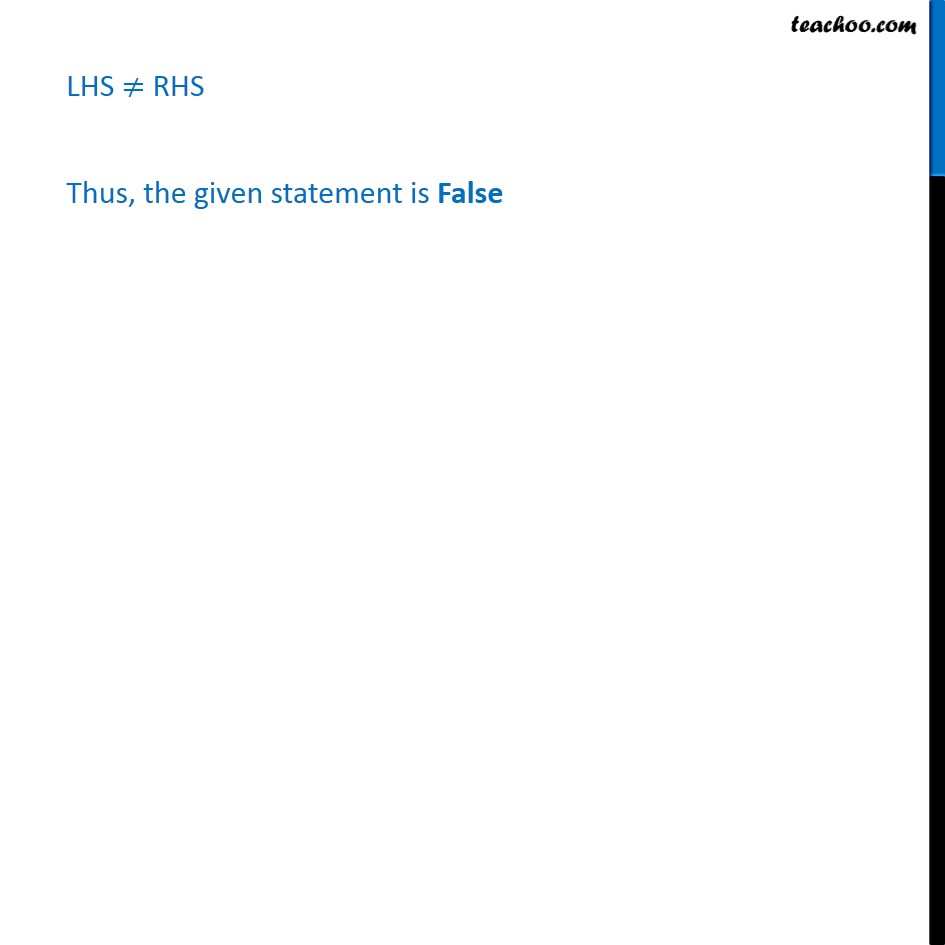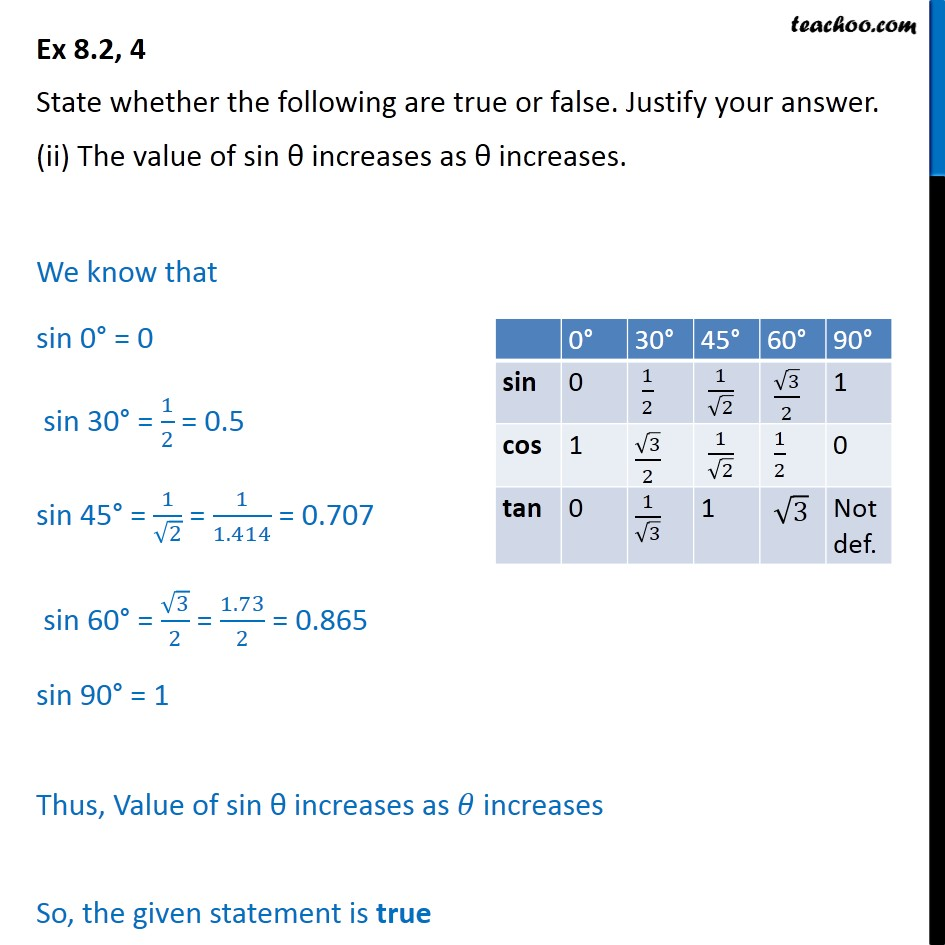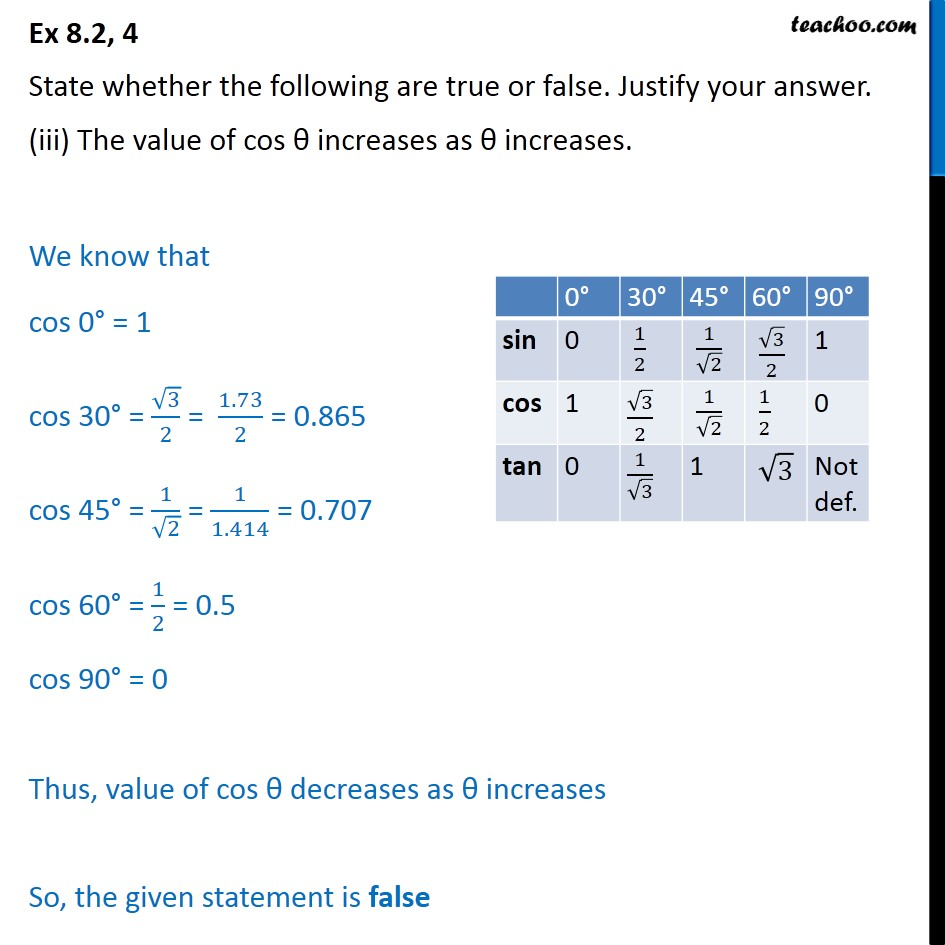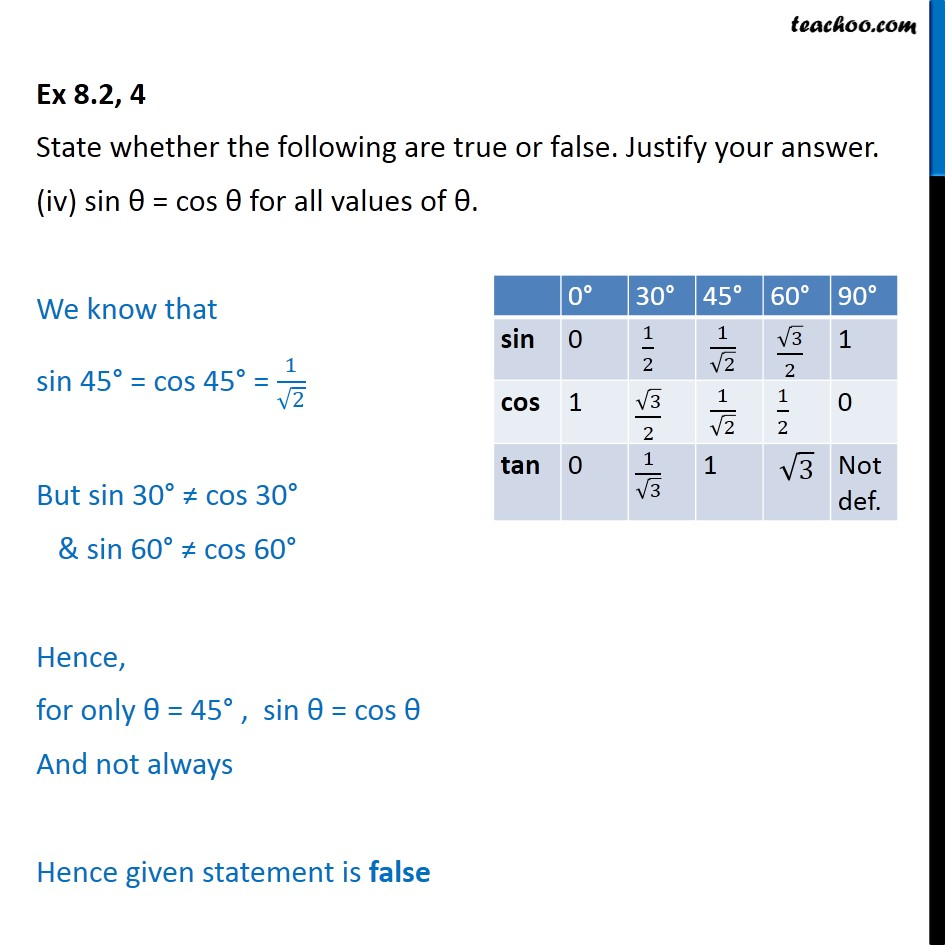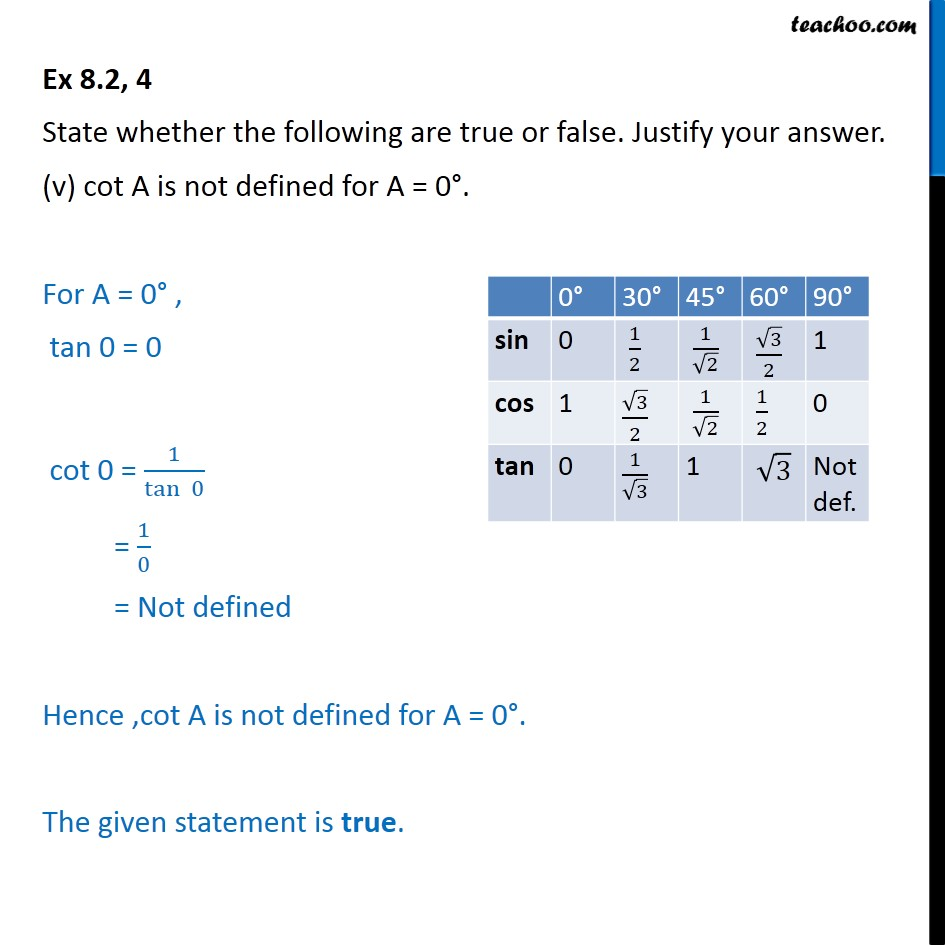Subscribe to our Youtube Channel - https://you.tube/teachoo

1. Chapter 8 Class 10 Introduction to Trignometry
2. Serial order wise
3. Ex 8.2

Transcript

Ex 8.2, 4 State whether the following are true or false. Justify your answer. sin (A + B) = sin A + sin B. We have to prove sin (A + B) = sin A + sin B Assuming A = 60° & B = 30° LHS ≠ RHS Thus, the given statement is False Ex 8.2, 4 State whether the following are true or false. Justify your answer. (ii) The value of sin θ increases as θ increases. We know that sin 0° = 0 sin 30° = 1/2 = 0.5 sin 45° = 1/√2 = 1/1.414 = 0.707 sin 60° = √3/2 = 1.73/2 = 0.865 sin 90° = 1 Thus, Value of sin θ increases as 𝜃 increases So, the given statement is true Ex 8.2, 4 State whether the following are true or false. Justify your answer. (iii) The value of cos θ increases as θ increases. We know that cos 0° = 1 cos 30° = √3/2 = 1.73/2 = 0.865 cos 45° = 1/√2 = 1/1.414 = 0.707 cos 60° = 1/2 = 0.5 cos 90° = 0 Thus, value of cos θ decreases as θ increases So, the given statement is false Ex 8.2, 4 State whether the following are true or false. Justify your answer. (iv) sin θ = cos θ for all values of θ. We know that sin 45° = cos 45° = 1/√2 But sin 30° ≠ cos 30° & sin 60° ≠ cos 60° Hence, for only θ = 45° , sin θ = cos θ And not always Hence given statement is false Ex 8.2, 4 State whether the following are true or false. Justify your answer. (v) cot A is not defined for A = 0°. For A = 0° , tan 0 = 0 cot 0 = 1/(tan⁡ 0) = 1/0 = Not defined Hence ,cot A is not defined for A = 0°. The given statement is true.

Ex 8.2